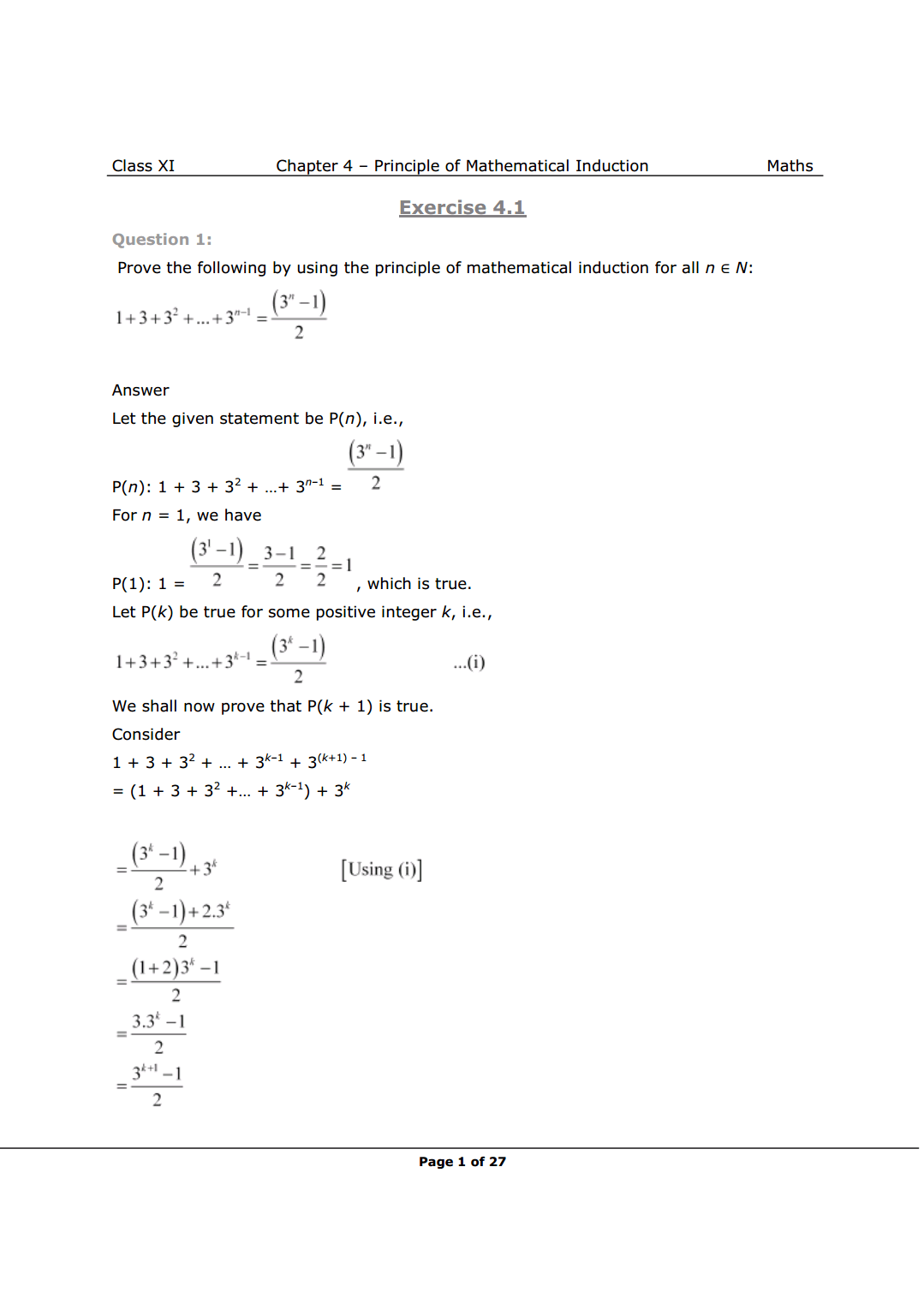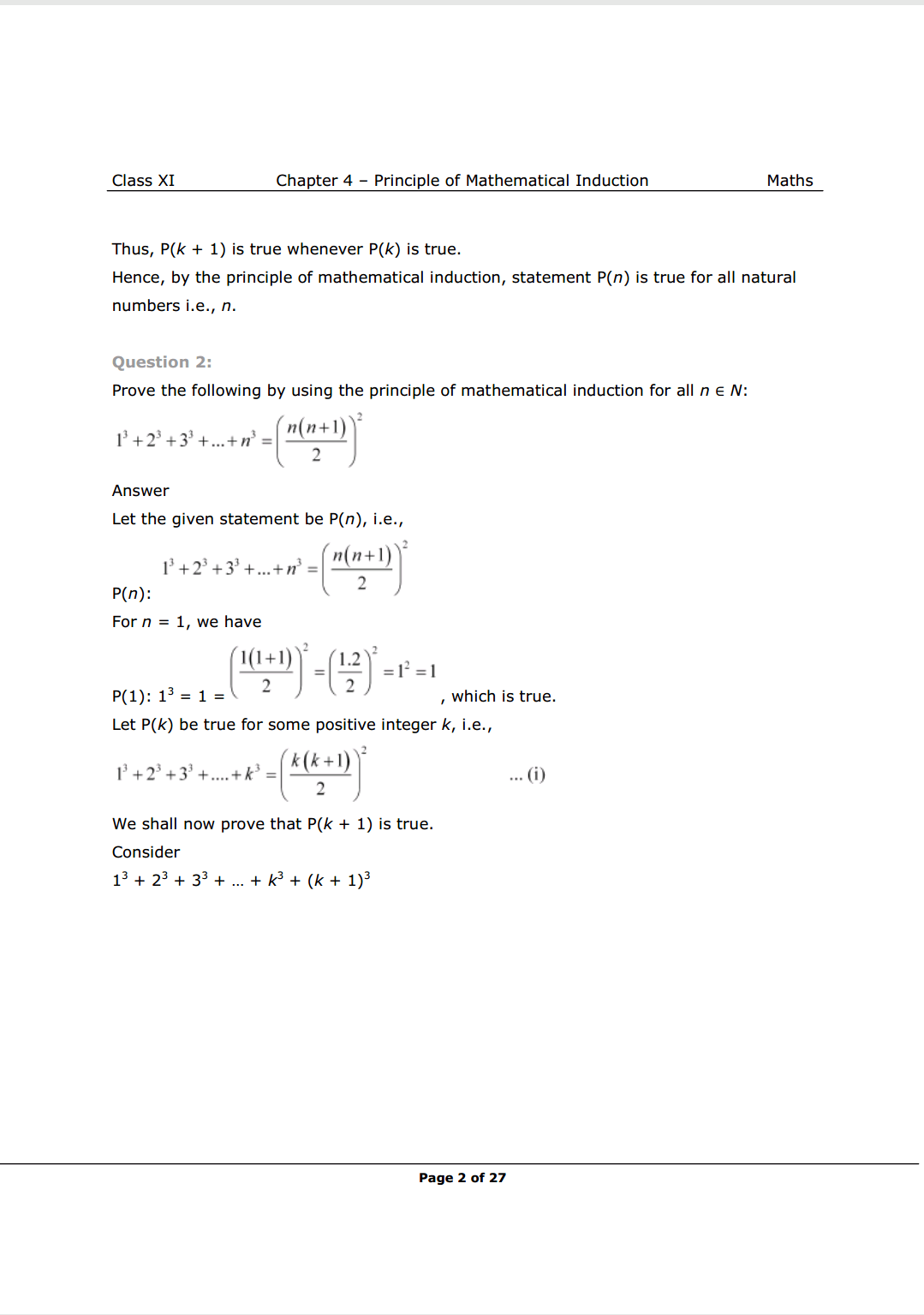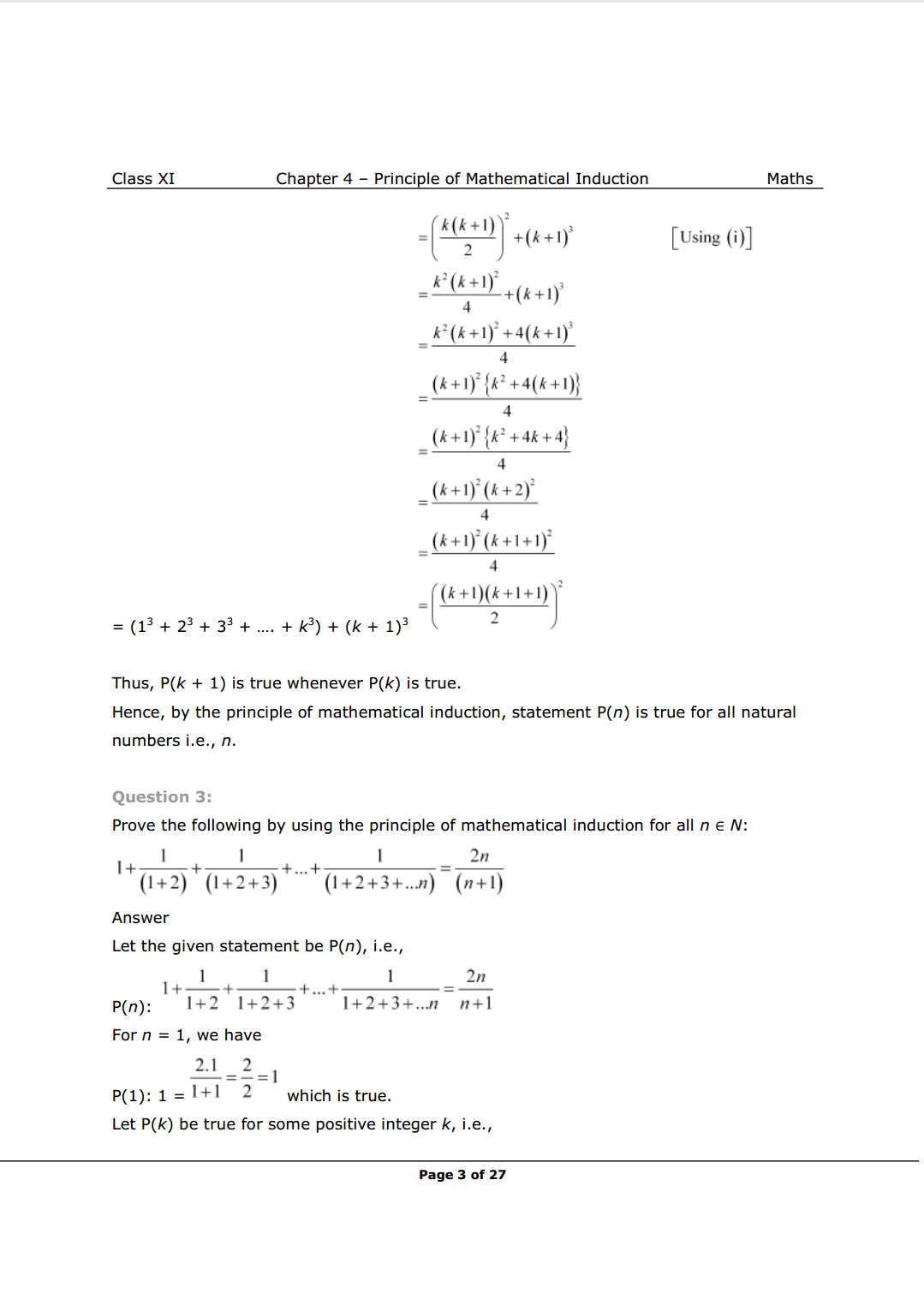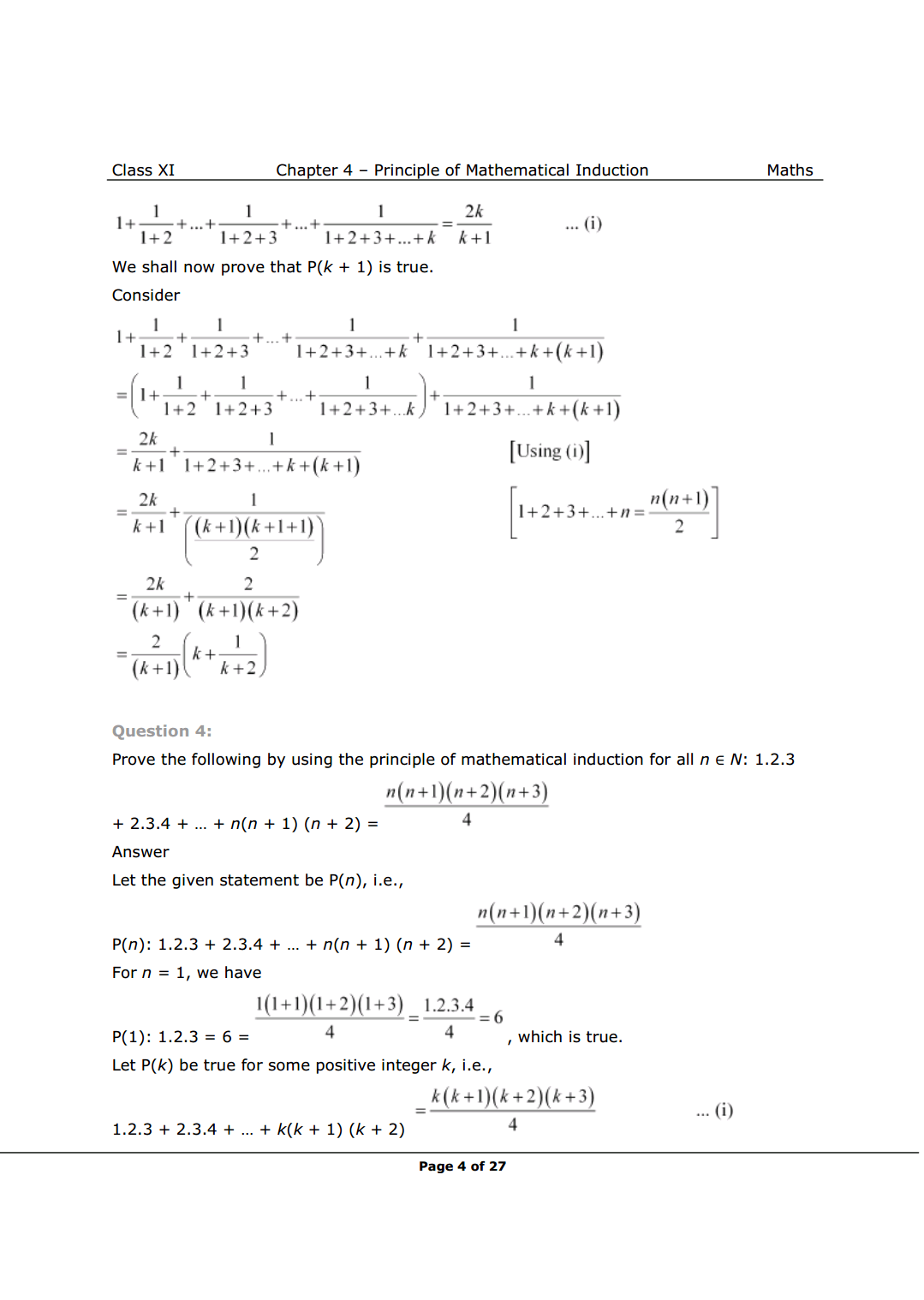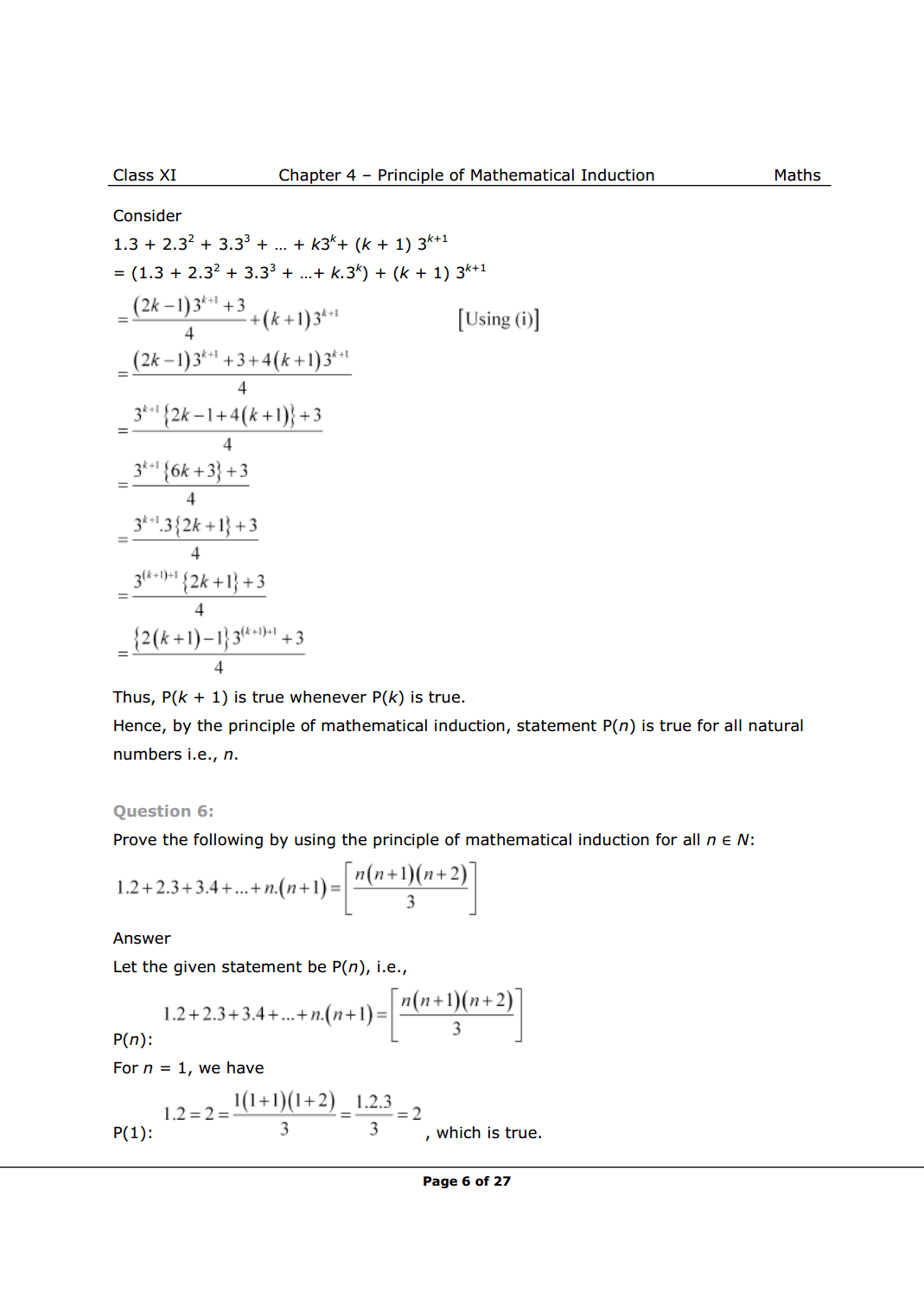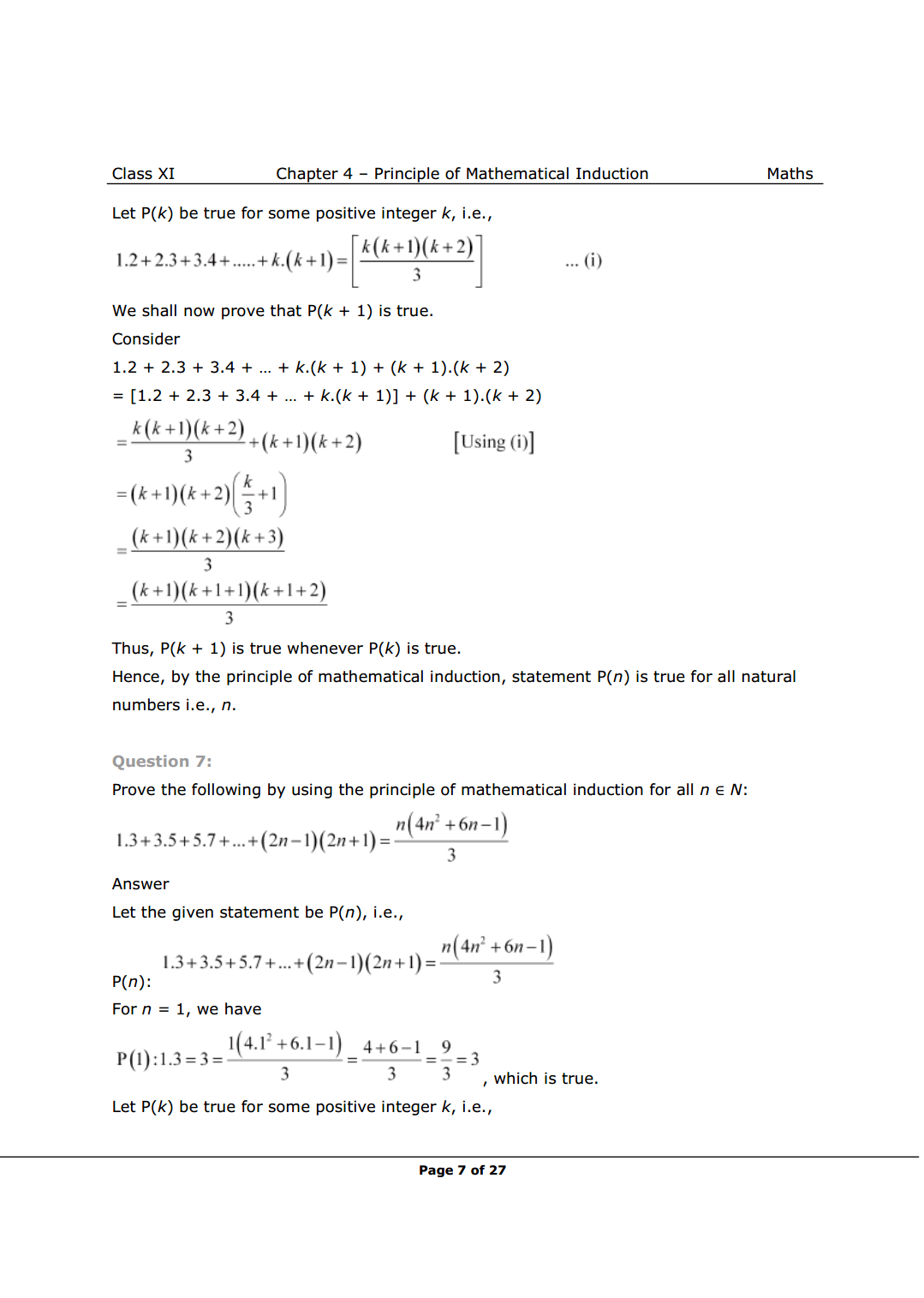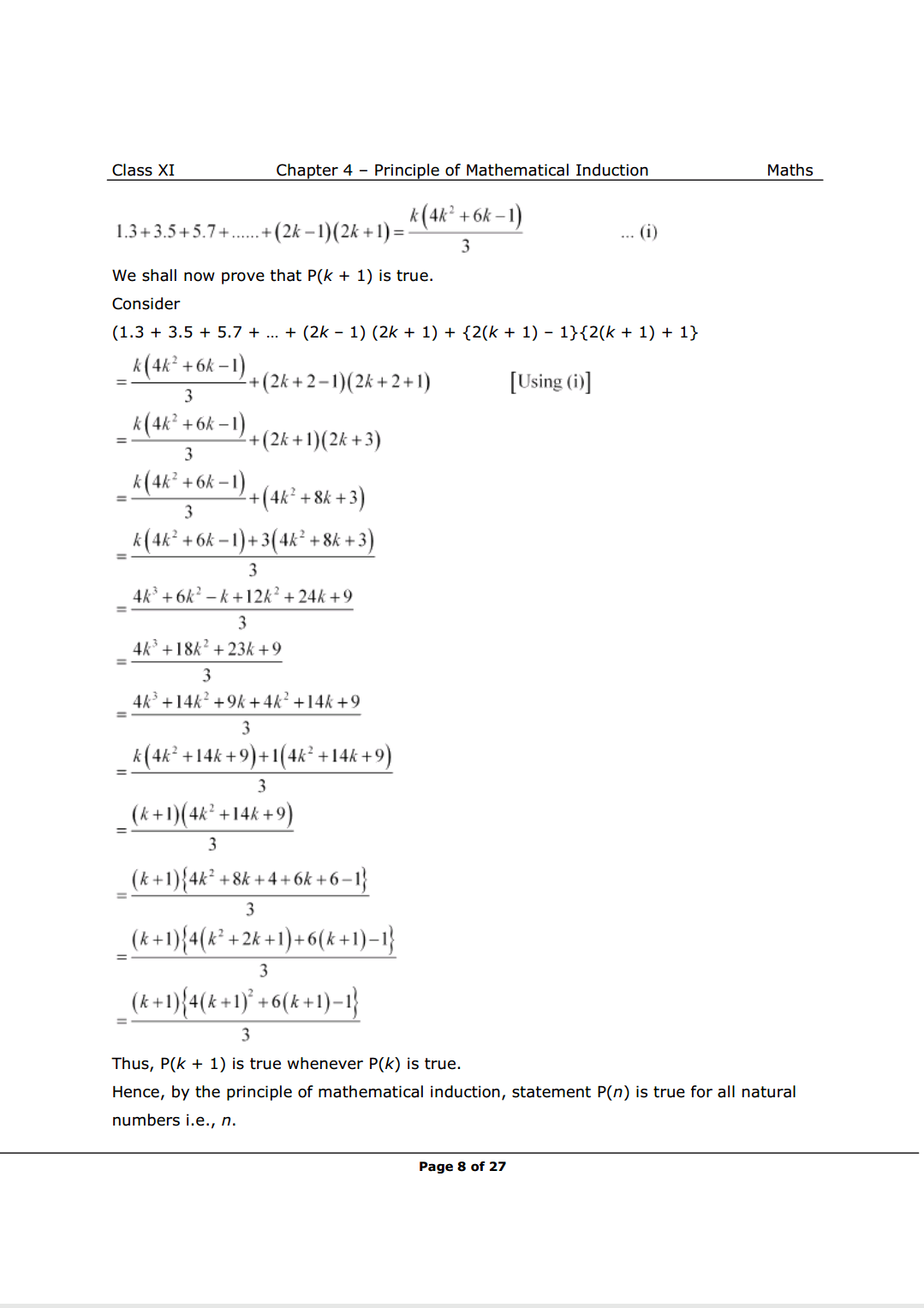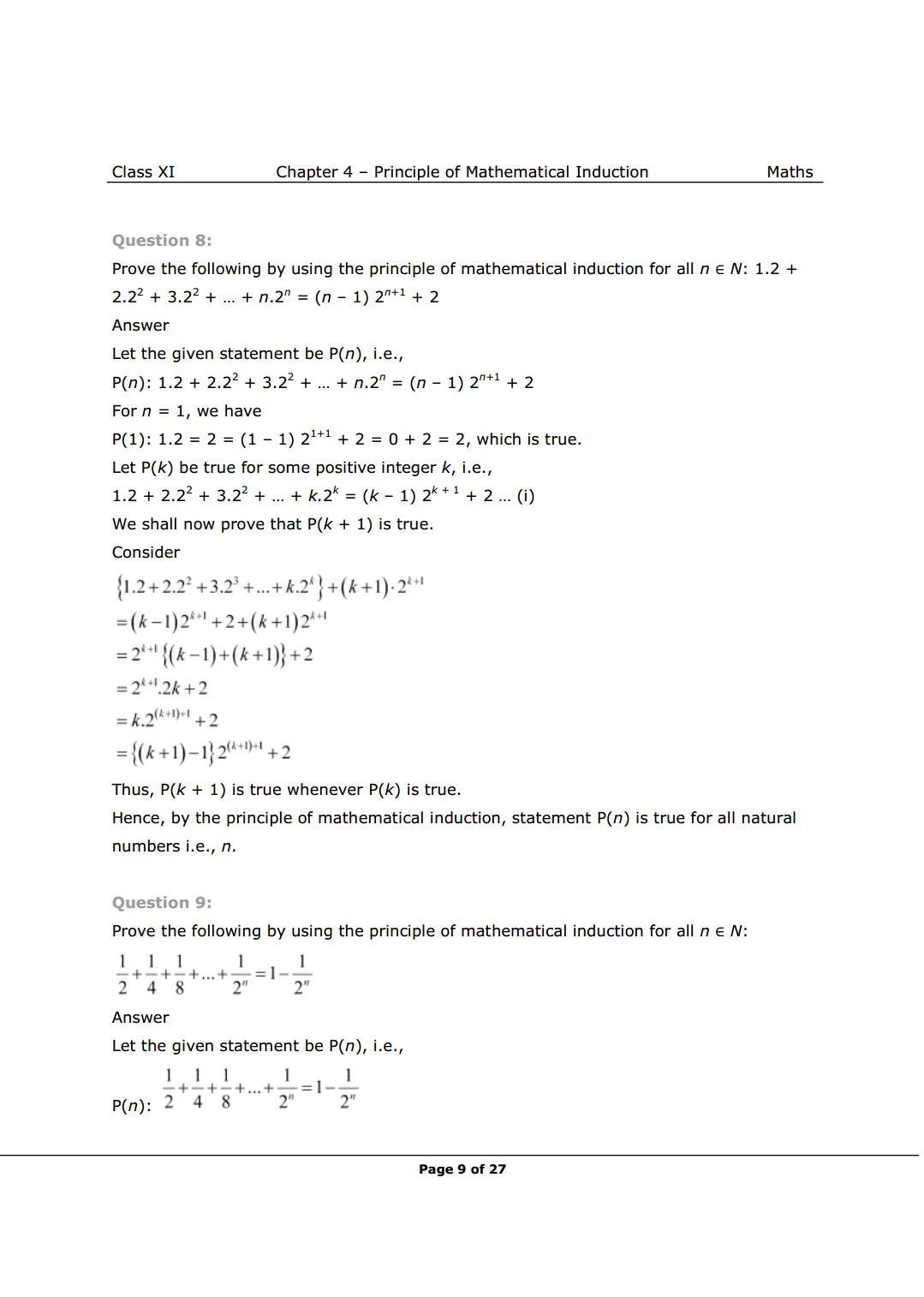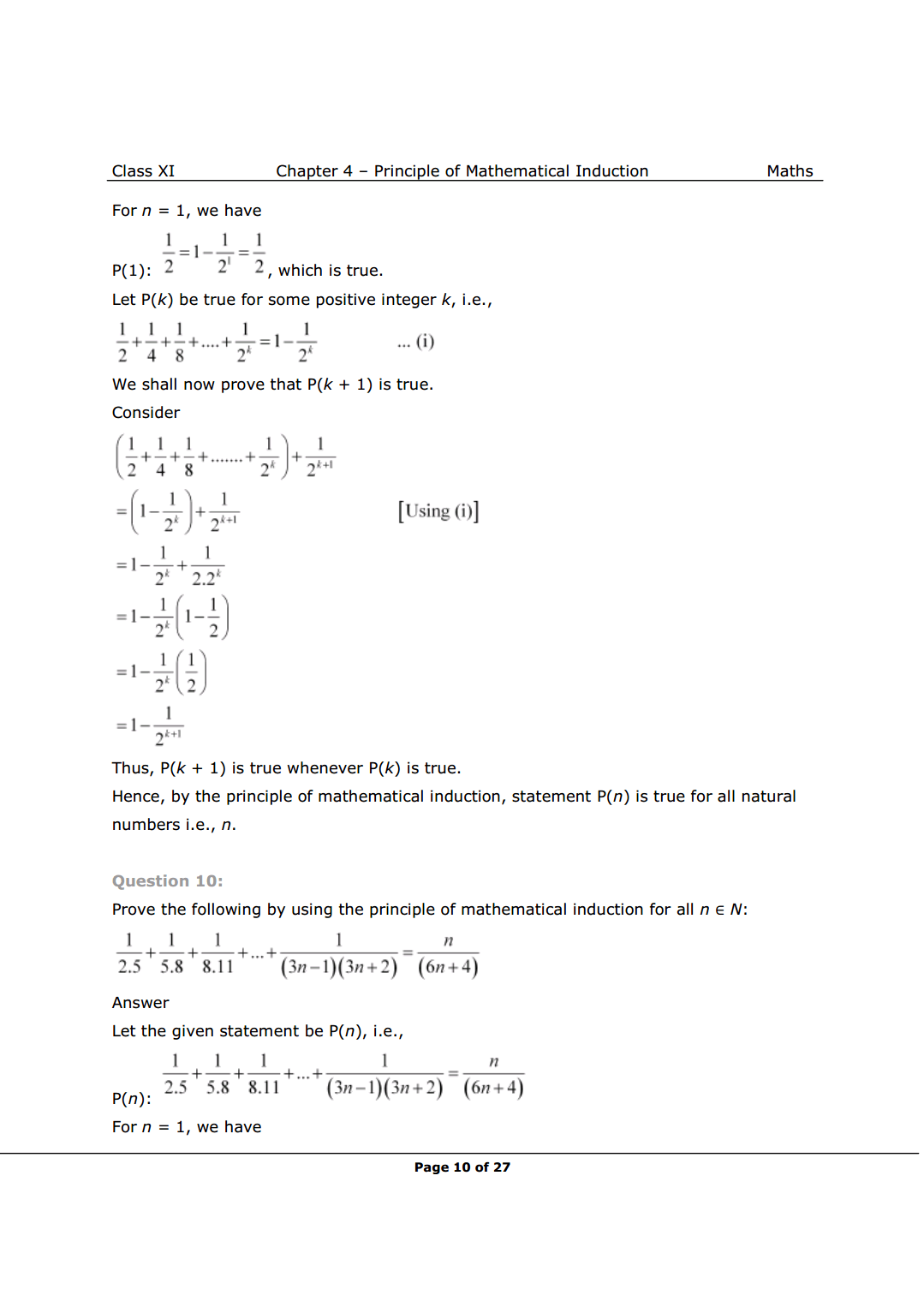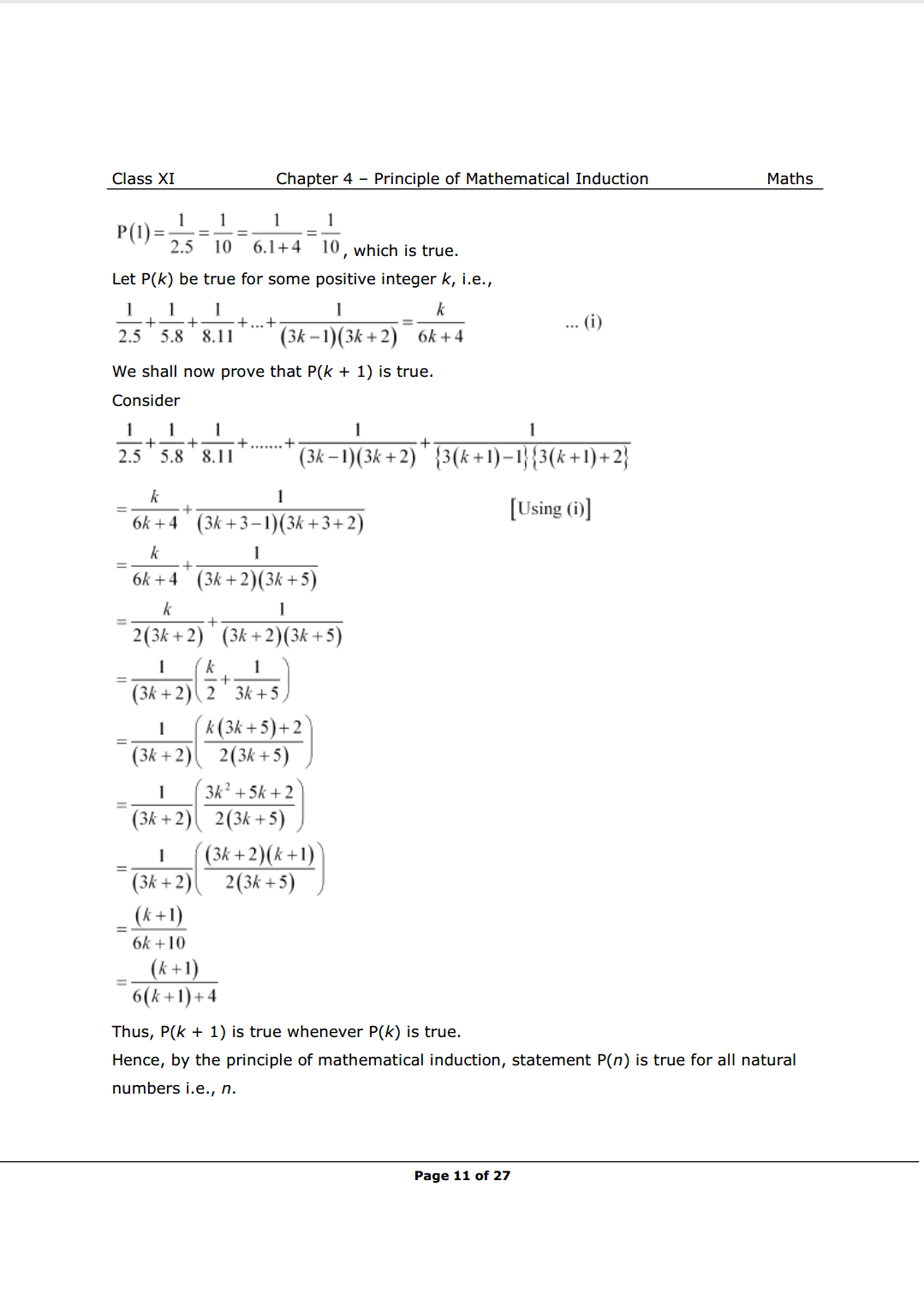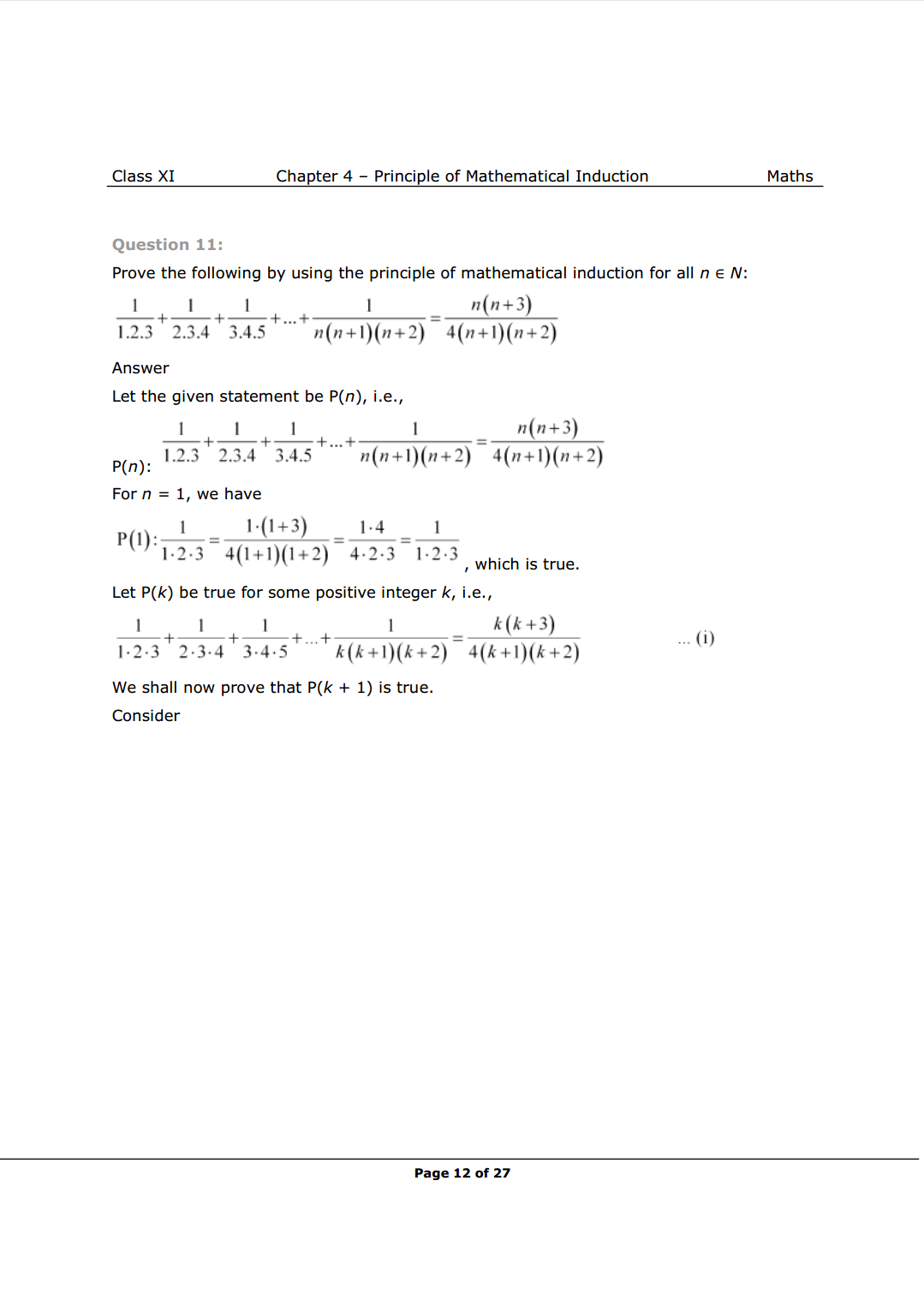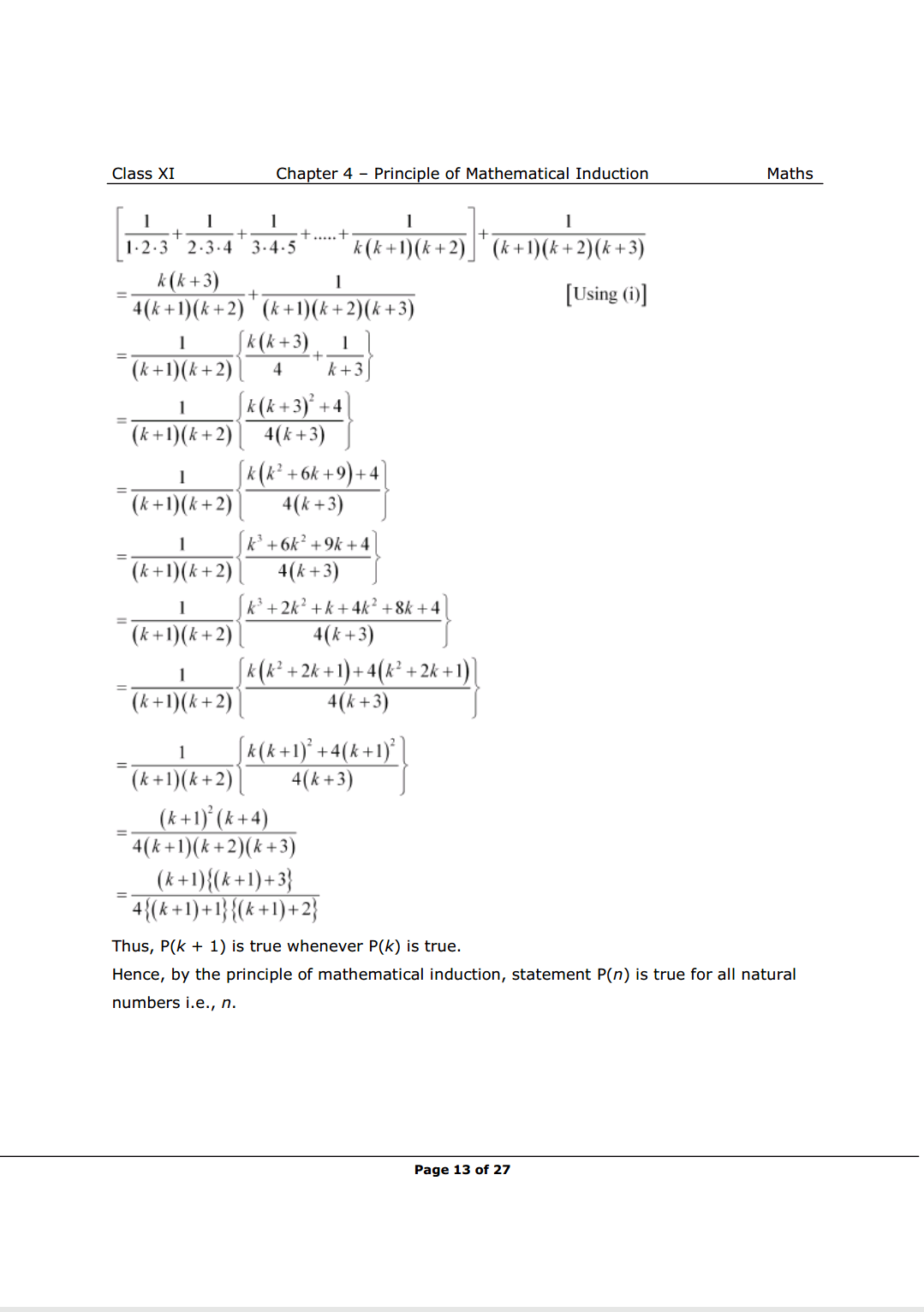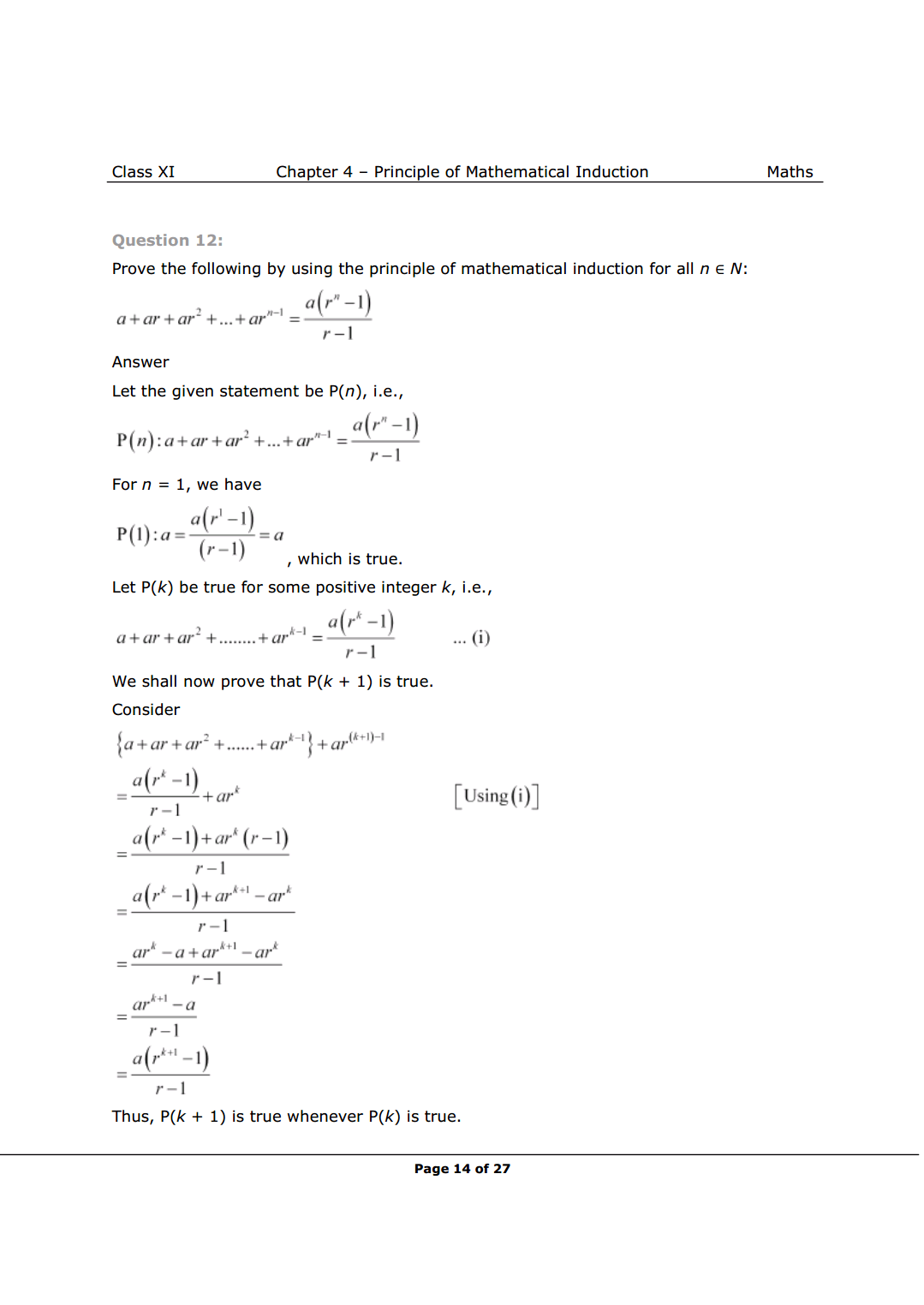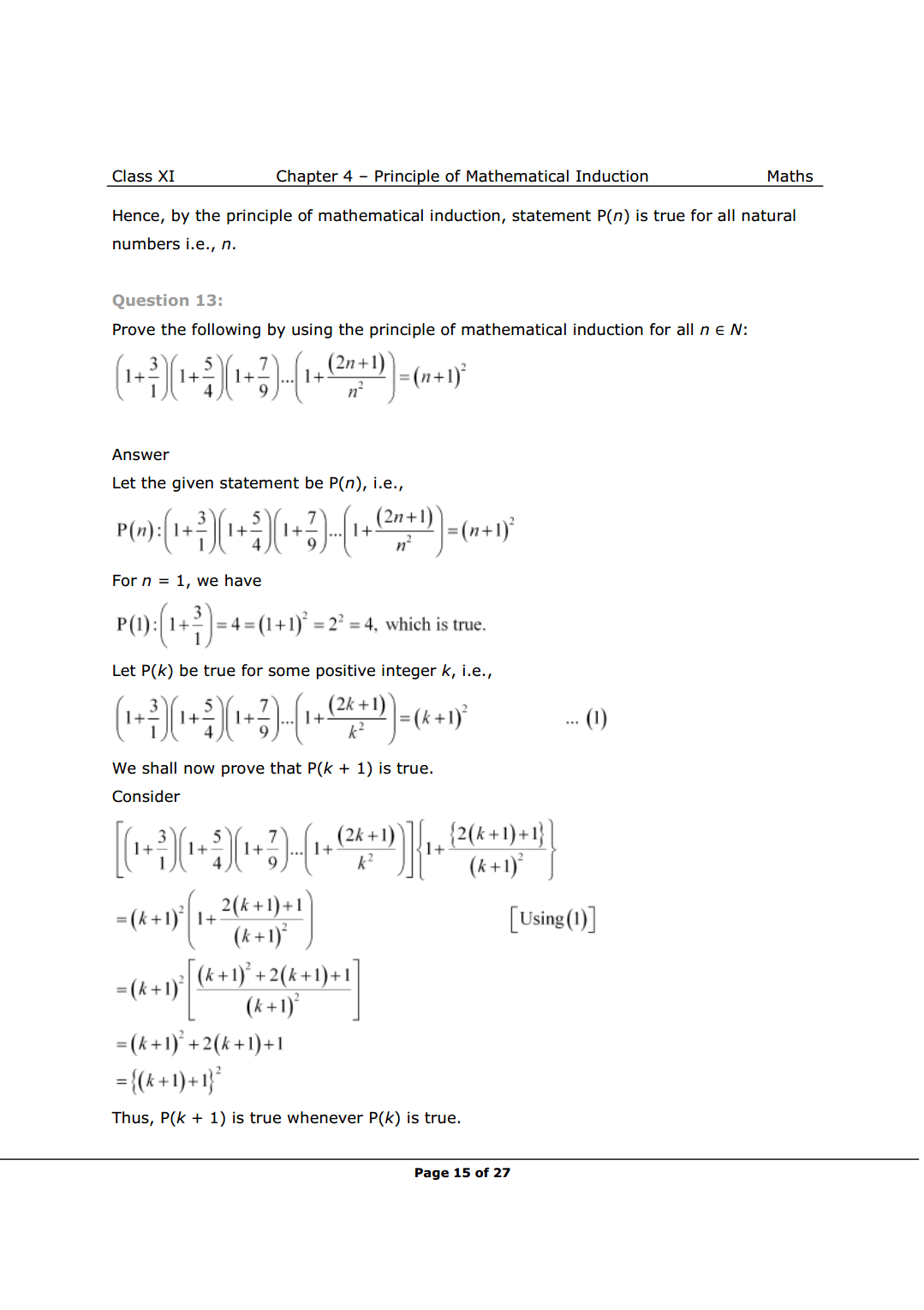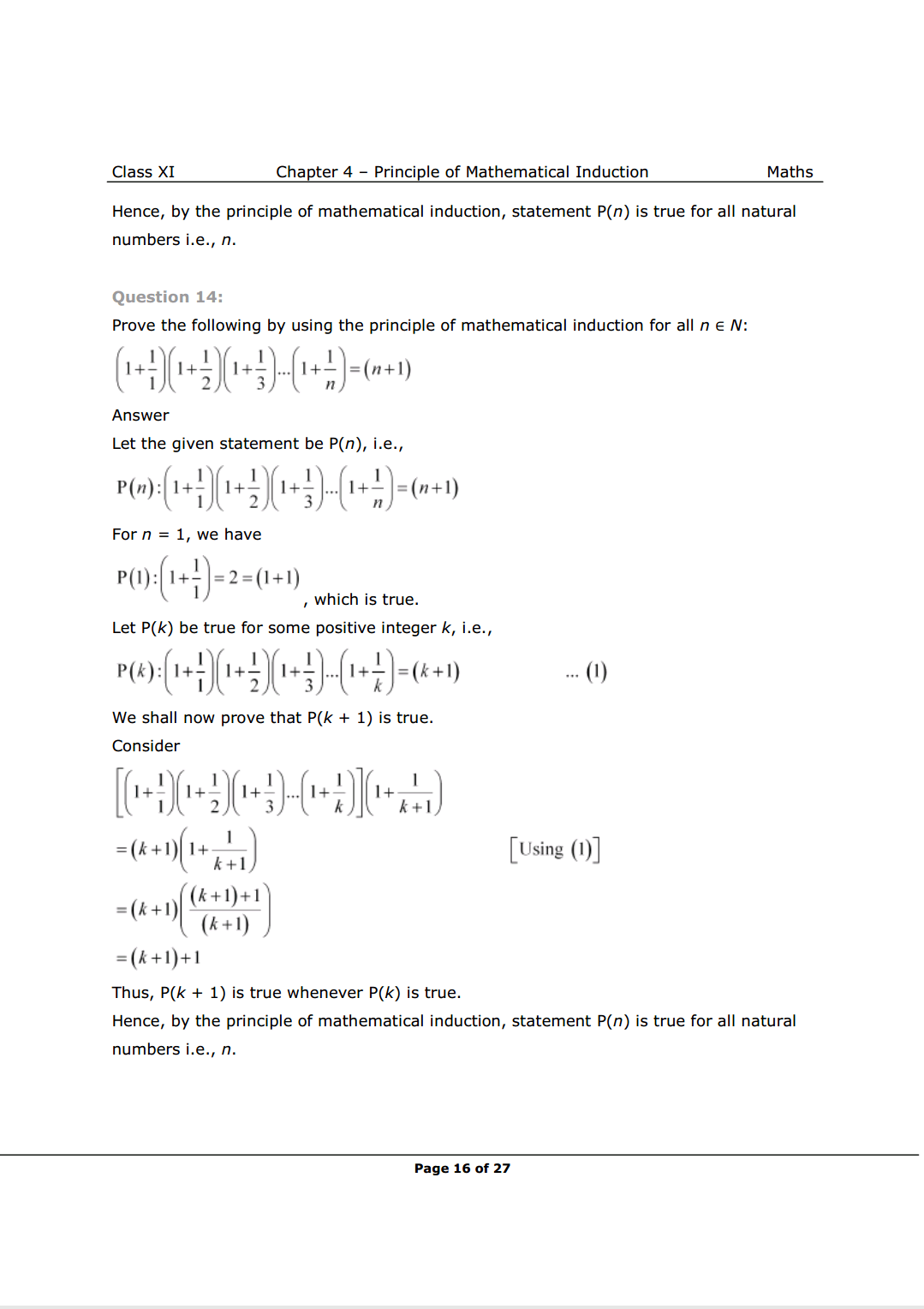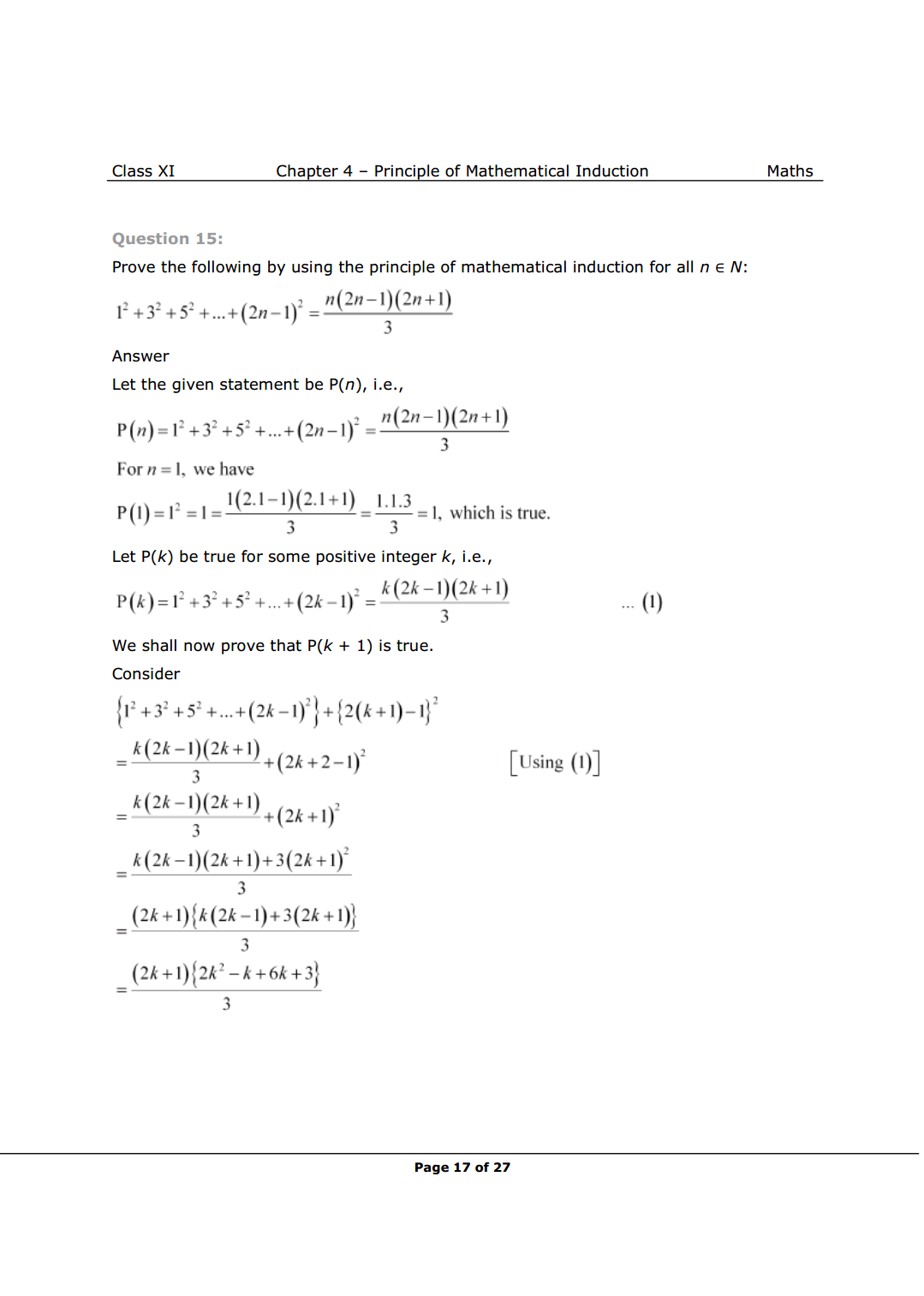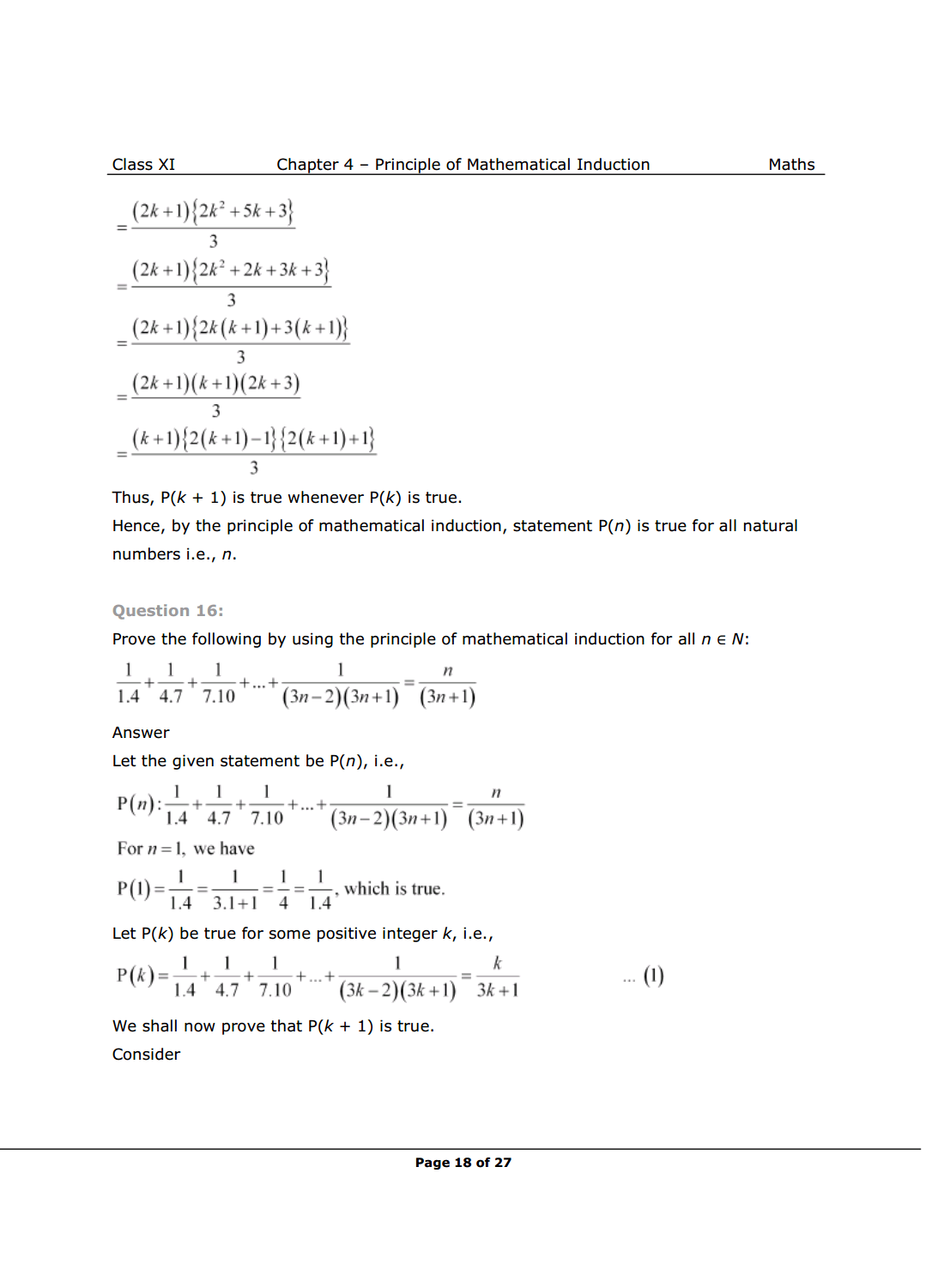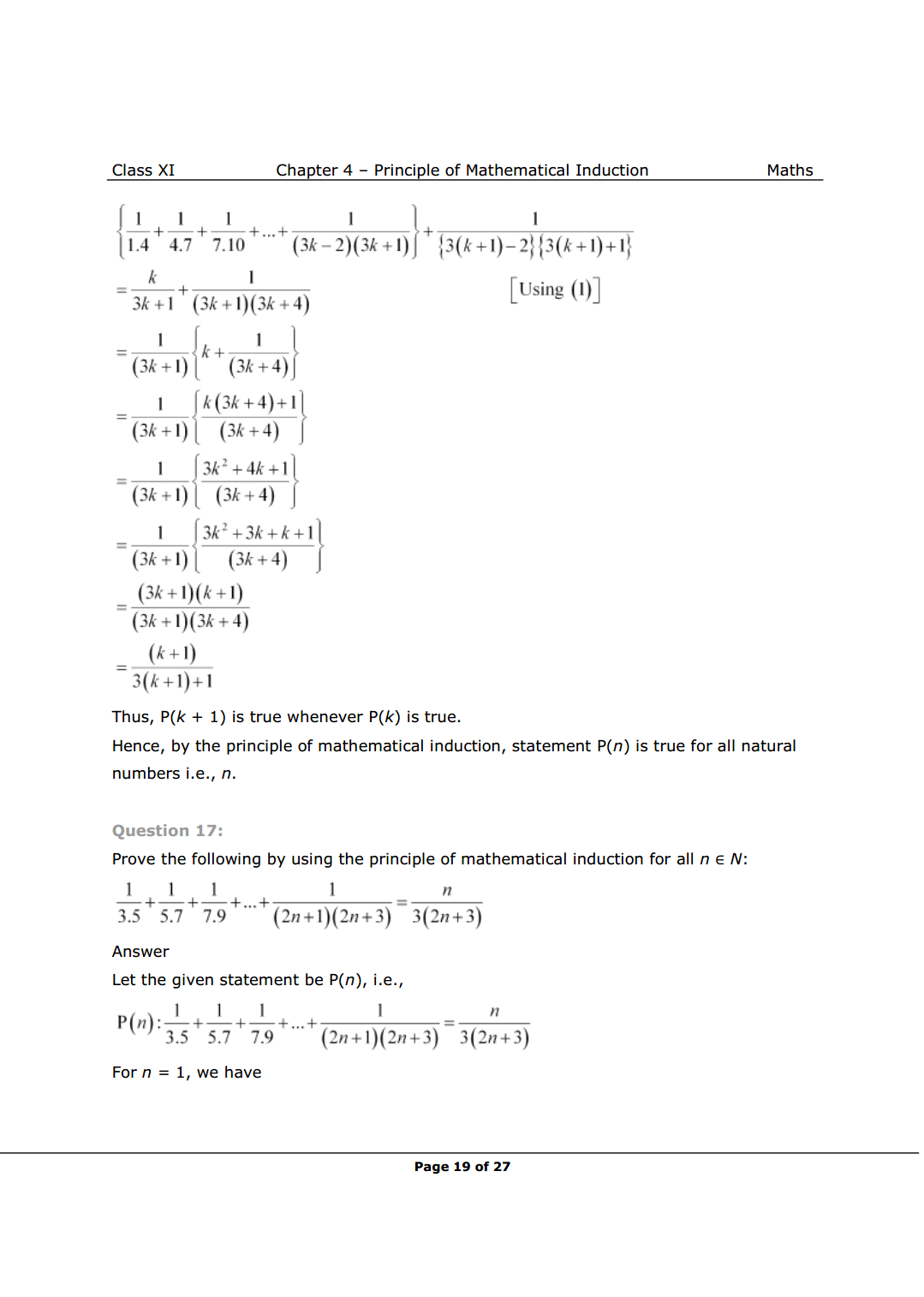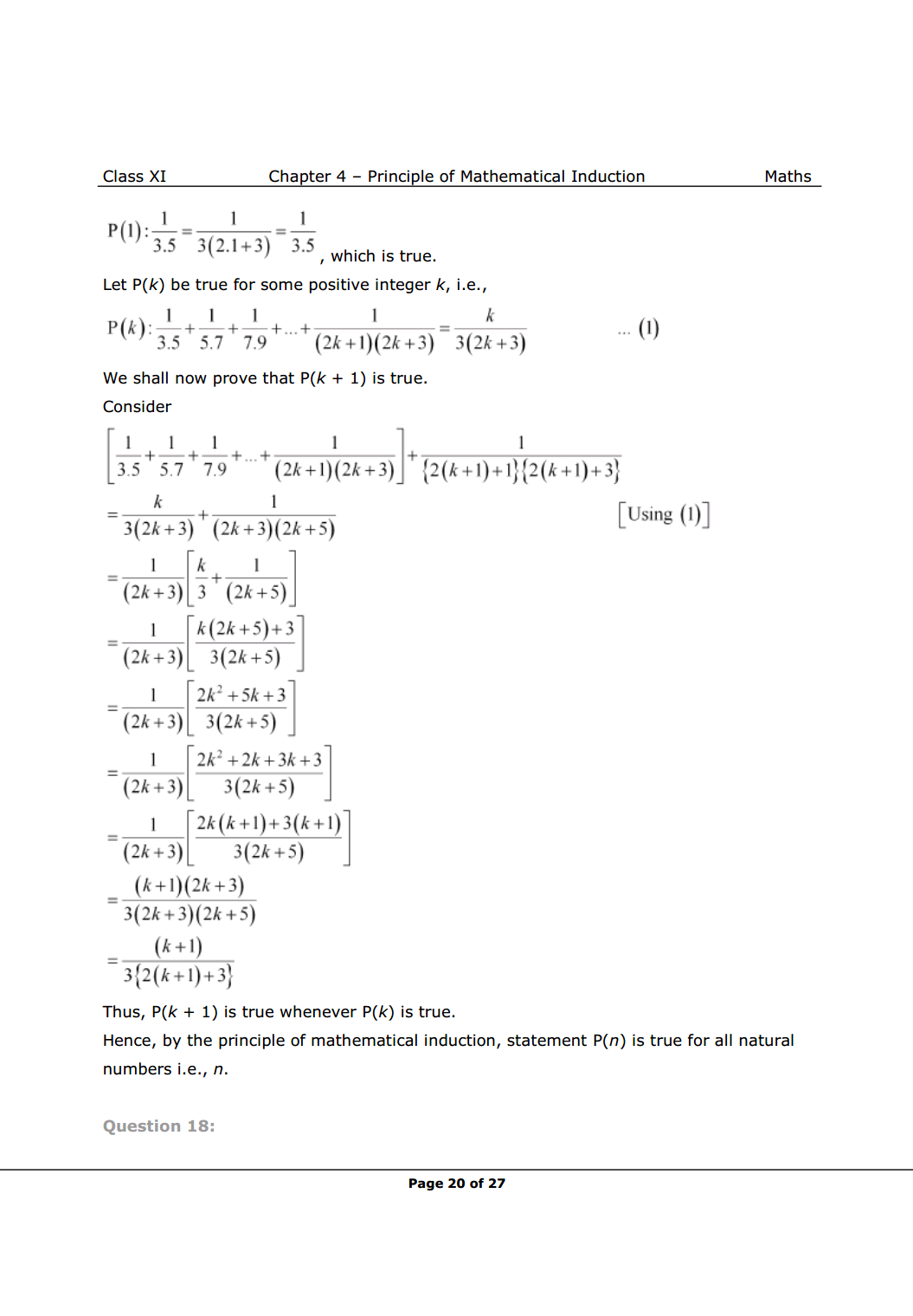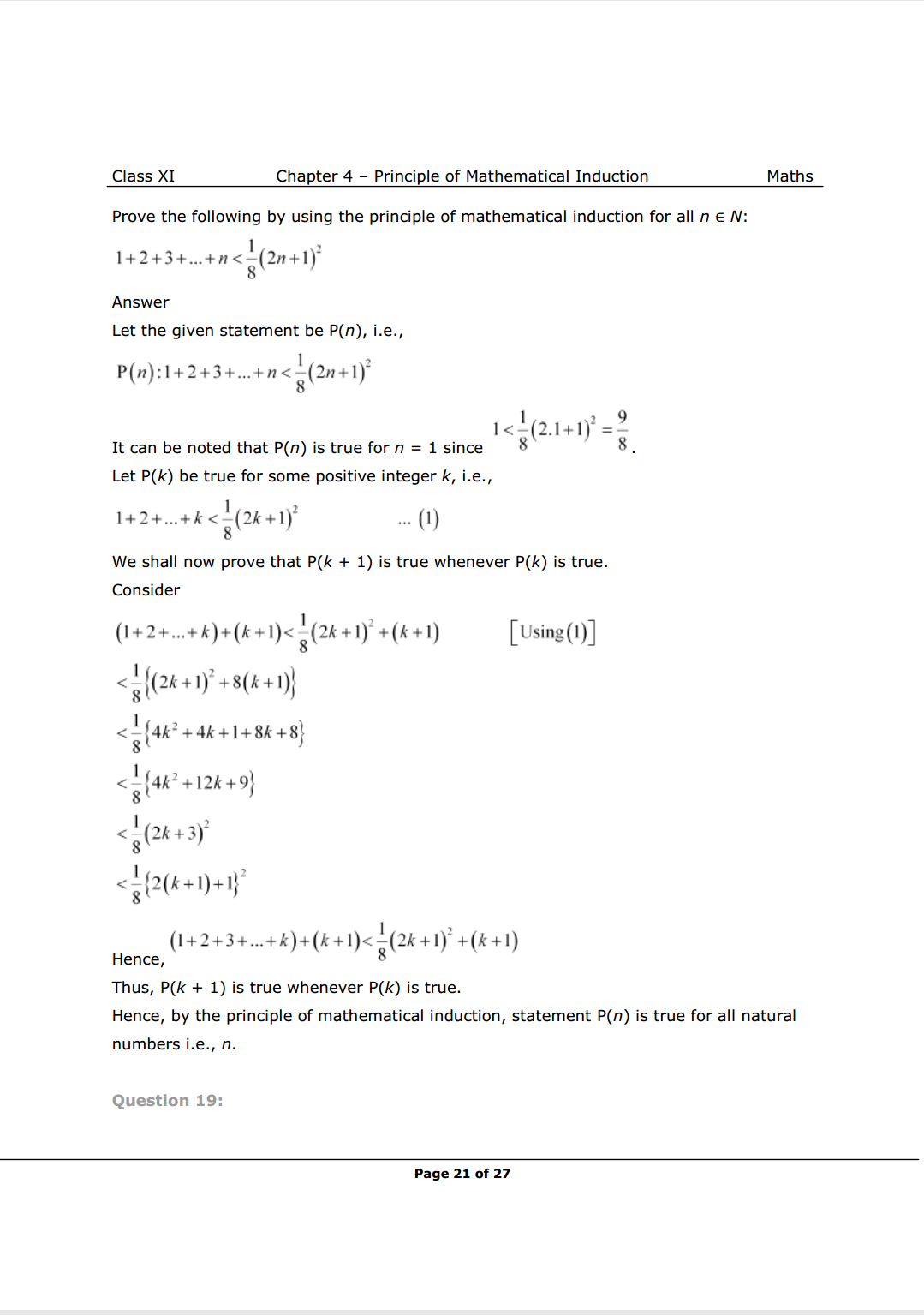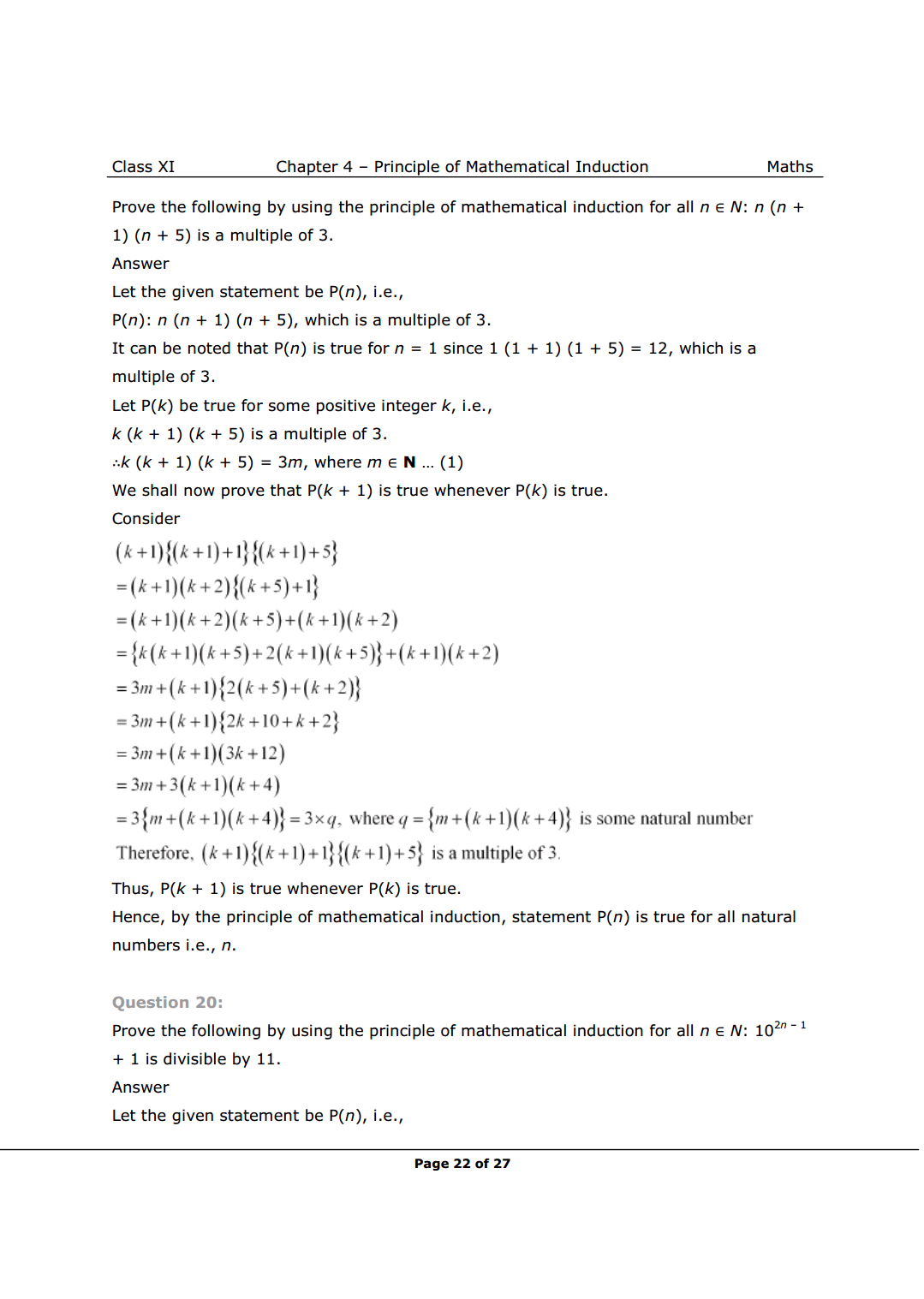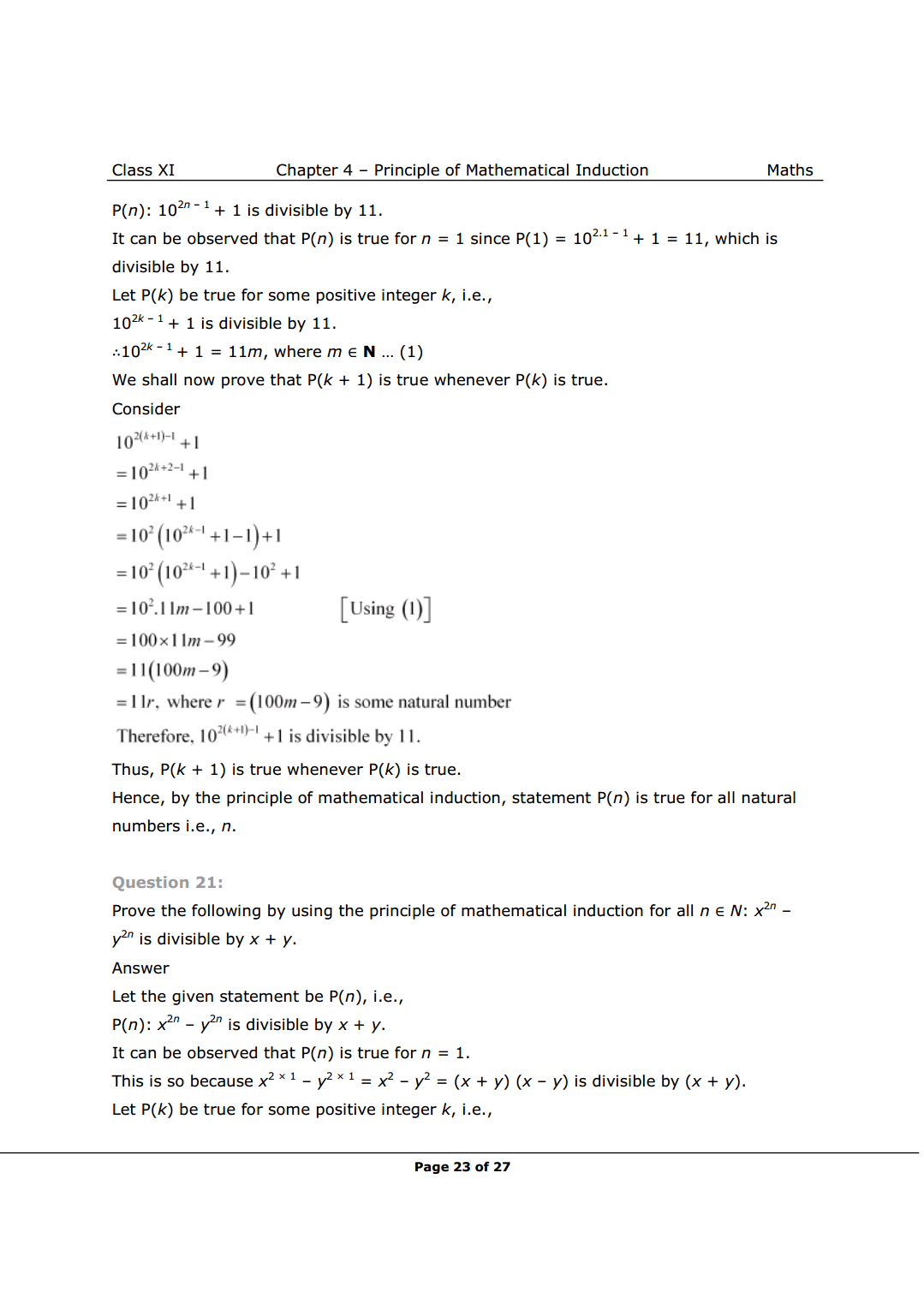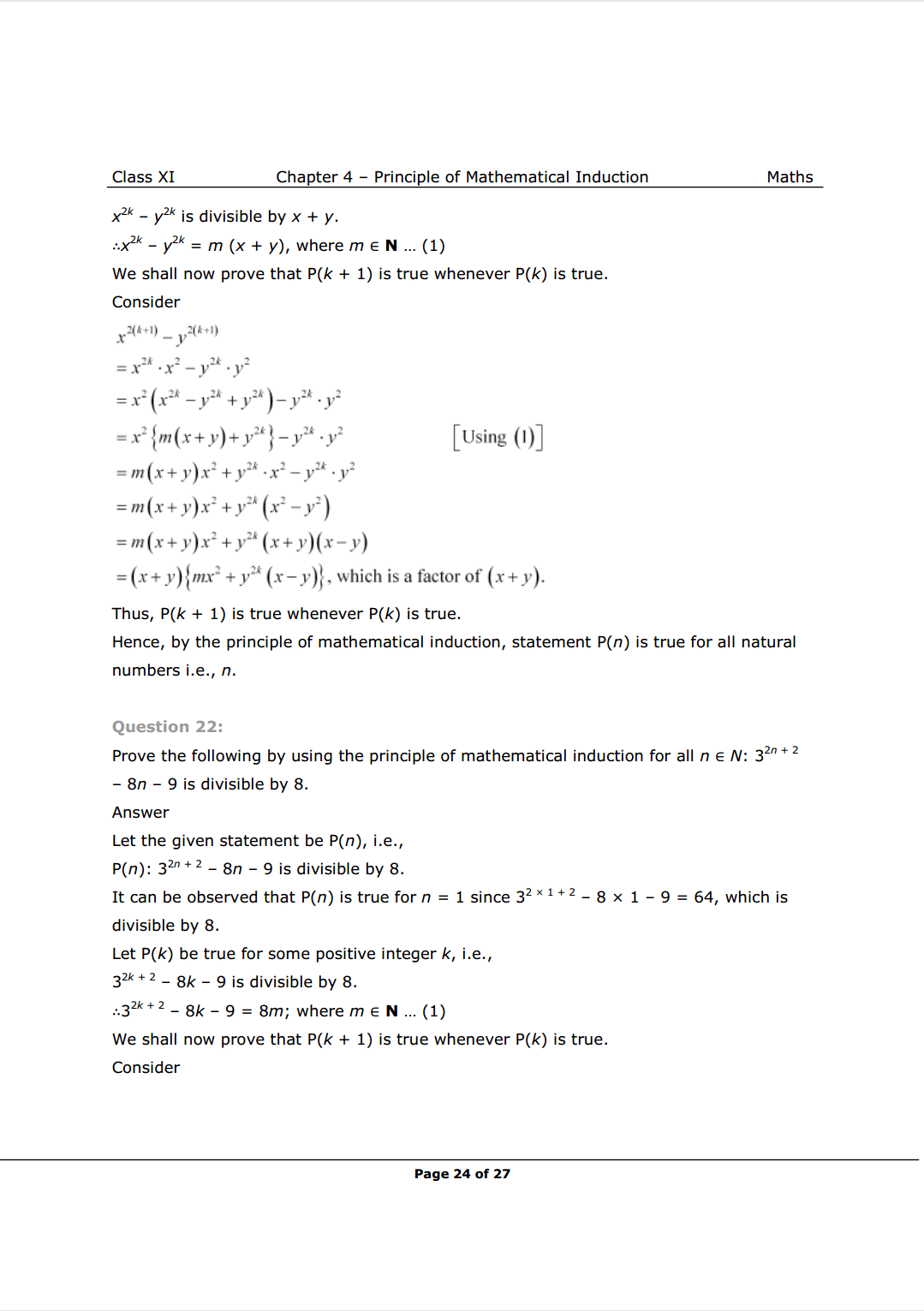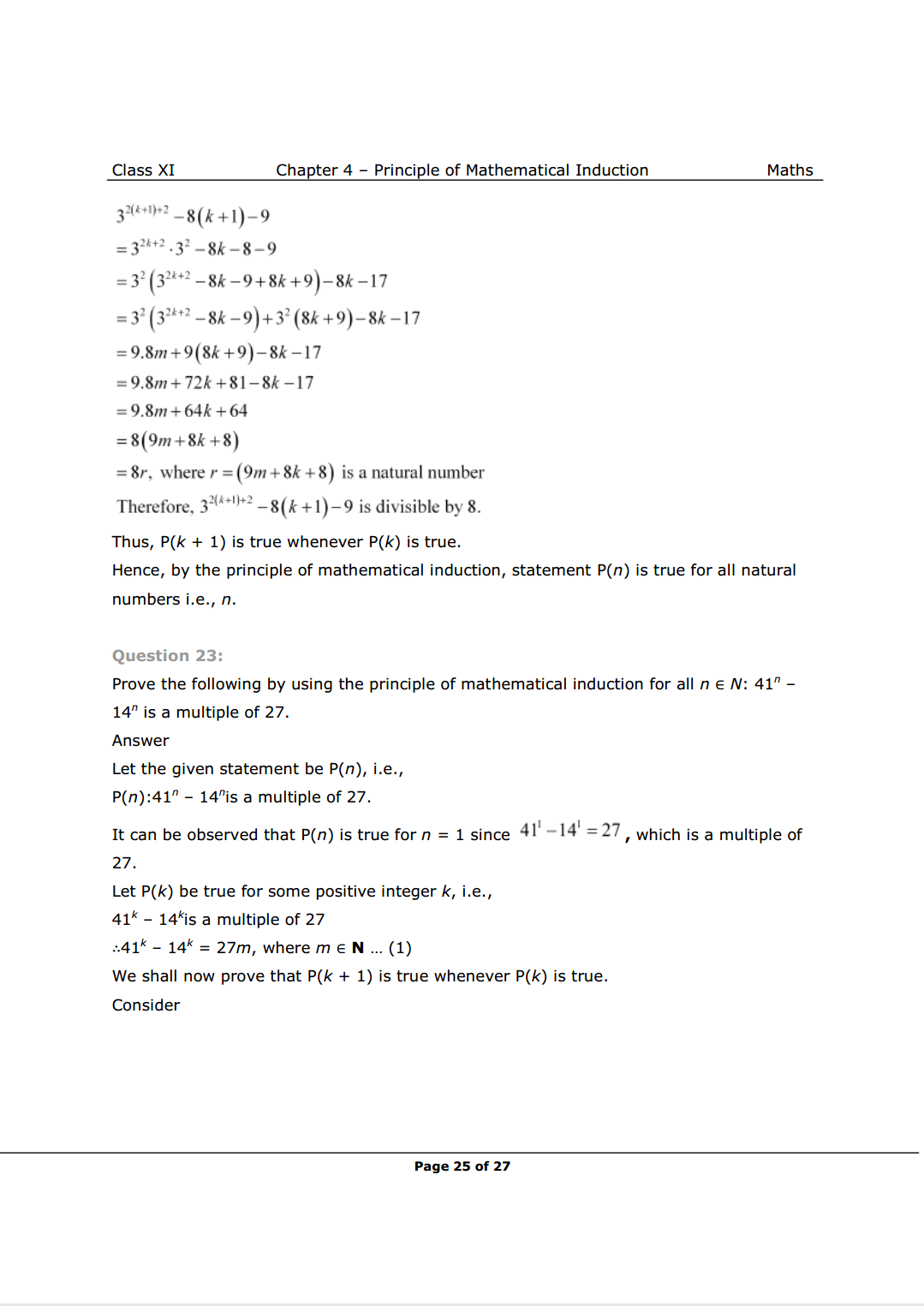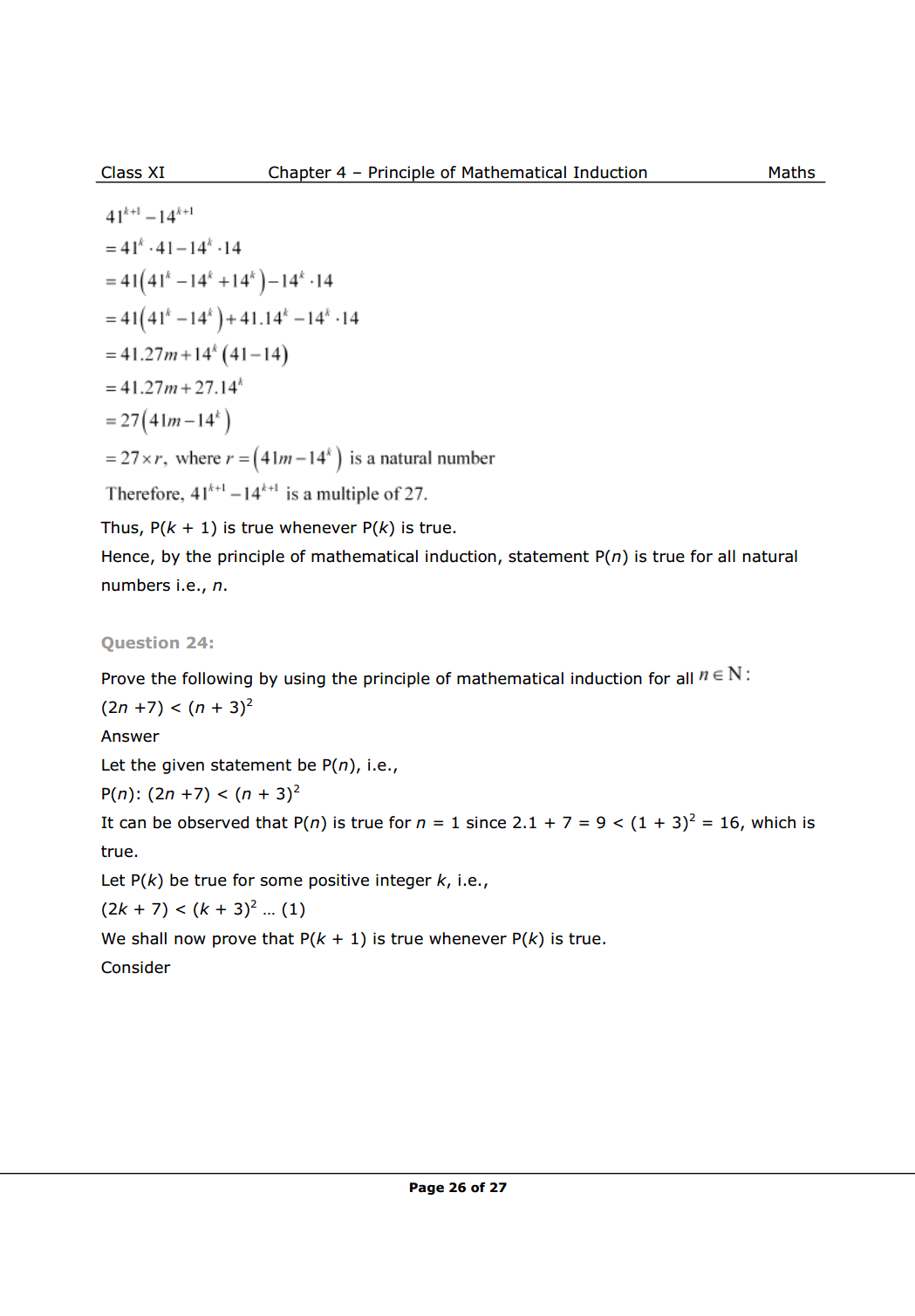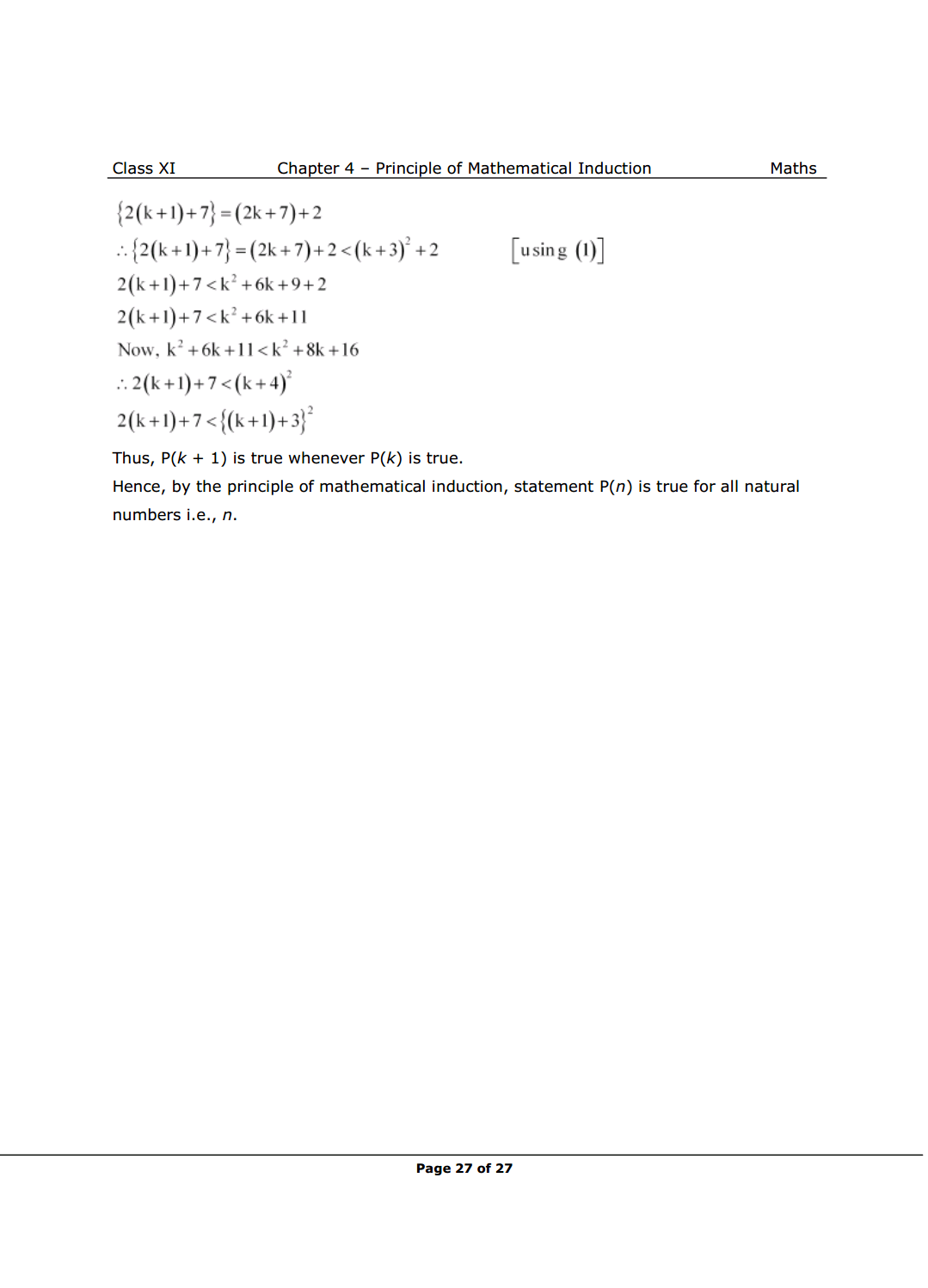Most Affordable JEE | NEET | 8,9,10 Preparation by Kota's Top IITian Doctor Faculties

# NCERT Solutions for Class 11 Maths chapter 4 - Principle of Mathematical Induction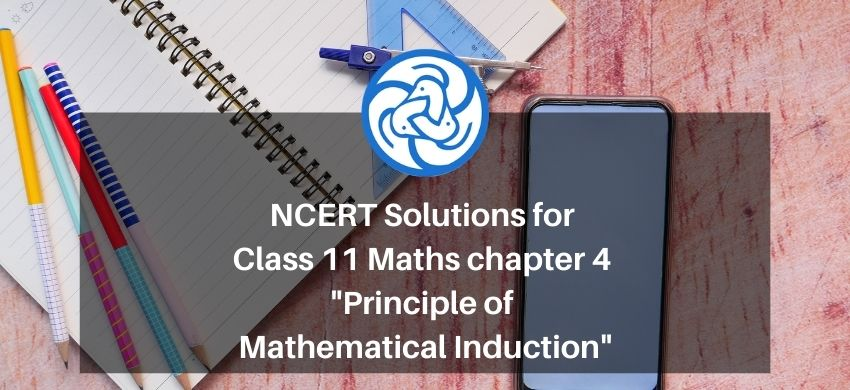Hey, are you a class 11 student and looking for ways to download NCERT Solutions for Class 11 Maths chapter 4 "Principle of Mathematical Induction"? If yes. Then read this post till the end.

In this article, we have listed NCERT solutions for class 11 Math Chapter 4 in PDF that is prepared by Kota’s top IITian’s Faculties by keeping Simplicity in mind.

If you want to learn and understand class 11 Maths Chapter 4 "Principle of Mathematical Induction" in an easy way then you can use these solutions PDF.

NCERT Solutions helps students to Practice important concepts of subjects easily. Class 11 Maths solutions provide detailed explanations of all the NCERT exercise questions that students can use to clear their doubts instantly.

If you want to score high in your class 11 Maths Exam then it is very important for you to have a good knowledge of all the important topics, so to learn and practice those topics you can use eSaral NCERT Solutions.

So, without wasting more time Let’s start.

### Download The PDF of NCERT Solutions for Class 11 Maths Chapter 4 "Principle of Mathematical Induction"